Next: Results Up: Dependence of single-particle energies Previous: Introduction

# Method

In the present study we consider EDF in the form given in Refs. [6,7],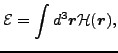(1)

where the energy density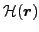can be represented as a sum of the kinetic energy and of the potential-energy isoscalar (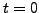) and isovector (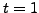) terms,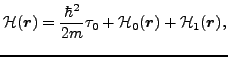(2)

which for the time-reversal and spherical symmetries imposed read: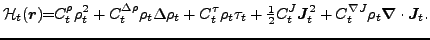(3)

Standard definitions of the local densities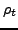,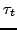, and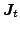were given in Refs. [8,7] and are not repeated here. Following the parametrization used for the Skyrme forces, we assume the dependence of the coupling parameters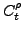on the isoscalar density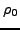as: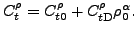(4)

Similarly as in Ref. , we note here that EDF of Eq. (1) depends linearly on twelve coupling constants,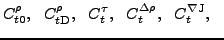and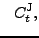(5)

forand 1. Therefore, due to the Hellmann-Feynman theorem , derivatives of the total energy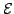with respect to the coupling constants are given by space integrals of densities appearing in Eq. (3) .

Variation of EDF in Eq. (1) with respect to the Kohn-Sham orbitals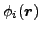, which define the local densities, gives the standard eigenequation,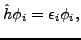(6)

where the Kohn-Sham one-body Hamiltonian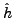is obtained as a functional derivative of EDF with respect to densities, see, e.g., Refs.[8,7,11]. It is obvious thatalso depends linearly on the coupling constants, and therefore, again due to the Hellmann-Feynman theorem, derivatives of the s.p. energies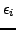with respect to the coupling constants are also given by space integrals of densities. As a consequence, we can expect that these derivatives are generic quantities weakly dependent on a particular parametrization of the functional, provided the functional has been adjusted to data and the underlying densities are correct.

The aim of the present work is not only to determine these derivatives but also to estimate to which extent they are generic. Of course, for a given set of coupling constants, they can be calculated from the Hellmann-Feynman theorem. Equivalently, their determination may rely on numerically calculating functions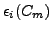, where index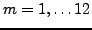is assumed to enumerate the twelve coupling constants (5). One simple option would be to calculate them from the finite-difference formula, for example as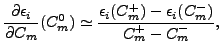(7)

with values of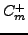and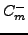suitably close to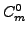. Had the functionsbeen exactly linear, the Hellmann-Feynman and finite-difference methods would have given exactly the same answers. If we aim at determining the degree to which they are not linear we have to proceed in another way.

To this end, the method of choice is the linear regression analysis , which makes a hypothesis of linearity and tests it by determining the regression coefficients and their standard deviations. In our case, we write the expression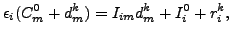(8)

where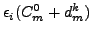are the s.p. energies calculated self-consistently for coupling constants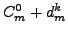that differ fromby suitably small numbers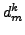. Index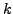enumerates different choices of these small numbers (which sample the vicinity of) and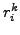are the residuals between the self-consistent results and linear approximation given by the regression coefficients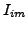and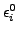. The regression method minimizes the residuals by adjusting the regression coefficients and determines their standard deviations. It is obvious that regression coefficientsconstitute estimates of derivatives (7), while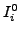are very close to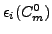.

The results below were obtained by using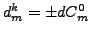, where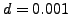to 0.005, depending on the Skyrme functional and nucleus, i.e., each of the twelve coupling constants was raised or lowered by the same percent fraction. (In some cases, for vanishing coupling constants, appropriate absolute shifts were used.) As a result, in each case the regression analysis was done by using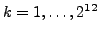=4096 samples.Next: Results Up: Dependence of single-particle energies Previous: Introduction
Jacek Dobaczewski 2008-05-18